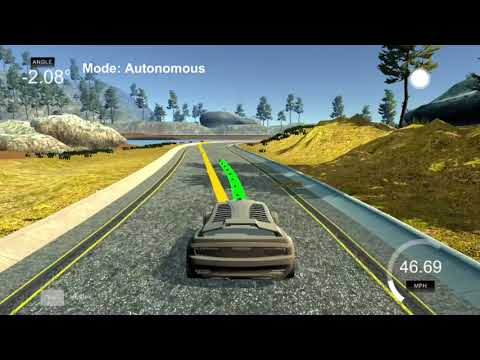# CarND-MPC-Project

View the Project on GitHub MarkBroerkens/CarND-MPC-Project# The Project

This project consists of a c++ implementation of a Model Predictive Control (MPC) to control the steering angle and the throttle acceleration of a car.# Compilation

1. Clone this repo.
2. Make a build directory: `mkdir build && cd build`
3. Compile: `cmake .. && make`
4. Run it: `./mpc`.

Note: I had to disable the line `options += "Numeric max_cpu_time 0.5\n";` in order to get predictions from the solver.

# Implementation

## The Model

The following euqations define rules for updating the prediction model at each time step.

``````x_[t+1] = x[t] + v[t] * cos(psi[t]) * dt
y_[t+1] = y[t] + v[t] * sin(psi[t]) * dt
psi_[t+1] = psi[t] + v[t] / Lf * delta[t] * dt
v_[t+1] = v[t] + a[t] * dt
cte[t+1] = f(x[t]) - y[t] + v[t] * sin(epsi[t]) * dt
epsi[t+1] = psi[t] - psides[t] + v[t] * delta[t] / Lf * dt
``````
• `x`, `y` position of the car in the coordinate system of the car
• `psi`orientation of the car
• `v` speed
• `cte`cross track error
• `èpsi` error with resperct to the orientation

• `Lf` measures the distance between the front of the vehicle and its center of gravity.
• `f(x)` is the evaluation of the polynomial `f` at point x
• and `psides` is the tangencial angle of the polynomial `f` evaluated at x.

## Timestep Length and Elapsed Duration (N & dt)

The prediction horizon is the duration over which predictions are made.

• `dt=100ms` the same duration as the latency of the system
• `N=11` this amount of steps allows prediction of 1.1s into the future. If the car would a max speed of 100mph we would predict the trajectory fo up to `49,17m = 100mph*0.44704mps/mph*1.1s = 100mph*1.1s` I reduced and increased the prediction horizon and figured out, that too many steps decreased the accuracy.

## Polynomial Fitting and MPC Preprocessing

The simulator returns the position of the car (x,y), its orientation (psi) and the waypoints (ptsx[i], ptsy[i]) in a global cordinate system. Before fitting the waypoints, they are translated into the coordinate system of the car:

``````for (int i = 0; i < ptsx.size(); i++) {
double dx = ptsx[i] - px;
double dy = ptsy[i] - py;
car_waypoints_x.push_back(dx * cos(psi) + dy * sin(psi)); // positive values: ahead
car_waypoints_y.push_back(dy * cos(psi) - dx * sin(psi)); // positive values: to the left
}
``````

After preprocessing, the polynomial is fitted using the helper function `polyfit` (file main.cpp at line 55). `

## Model Predictive Control with Latency

The goal of the Model Predictive Control algorithm is to find appropriate values for the steering angle and throttle that results in a predicted trajectory which is as close as possible to the polynom that was fitted to the waypoints

### Constraints

The actuators constraints limits the upper and lower bounds of the steering angle and throttle acceleration/brake.

``````// The upper and lower limits of delta are set to -25 and 25
const double steering_angle_constraint = 0.436332; // Constraint for steering angle
const double throttle_constraint = 1.0; // Constraint for acceleration

// delta of fist step is constrainted to the previous delta in order to consider the latency of 100ms
vars_lowerbound[delta_start] = prev_delta;
vars_upperbound[delta_start] = prev_delta;
for (int i = delta_start +1; i < a_start; i++) {
vars_lowerbound[i] = -steering_angle_constraint;
vars_upperbound[i] = steering_angle_constraint;
}

// acceleration of first step is constrained to the previous acceleration in order to consider the latency of 100ms
vars_lowerbound[a_start] = prev_throttle;
vars_upperbound[a_start] = prev_throttle;
for (int i = a_start +1; i < n_vars; i++) {
vars_lowerbound[i] = -throttle_constraint;
vars_upperbound[i] = throttle_constraint;
}
``````

### Costfunction

``````double ref_v = 25;
double ref_cte = 0;
double ref_epsi = 0;

const int cte_cost_weight = 50;
const int epsi_cost_weight = 5000;
const int v_cost_weight = 1000;
const int delta_cost_weight = 5;
const int a_cost_weight = 5;
const int high_speed_high_delta_penalty_weight = 20;
const int delta_change_cost_weight = 80000;
const int jerk_cost_weight = 10;

// The part of the cost based on the reference state.
for (int t = 0; t < N; t++) {
fg += cte_cost_weight * CppAD::pow(vars[cte_start + t] - ref_cte, 2);
fg += epsi_cost_weight * CppAD::pow(vars[epsi_start + t] - ref_epsi, 2);
fg += v_cost_weight * CppAD::pow(vars[v_start + t] - ref_v, 2);
}

// Minimize the use of actuators.
for (int t = 0; t < N - 1; t++) {
fg += delta_cost_weight * CppAD::pow(vars[delta_start + t], 2);
fg += a_cost_weight * CppAD::pow(vars[a_start + t], 2);
// penalty for high velocity with high delta
fg += high_speed_high_delta_penalty_weight * CppAD::pow(vars[a_start + t] * vars[v_start+t], 2);
}

// Minimize the value gap between sequential actuations.
for (int t = 0; t < N - 2; t++) {
fg += delta_change_cost_weight * CppAD::pow(vars[delta_start + t + 1] - vars[delta_start + t], 2);
fg += jerk_cost_weight * CppAD::pow(vars[a_start + t + 1] - vars[a_start + t], 2);
}
``````

Weights are added to each component of the cost function in order to tailor their impoact. An additional penalty is added in order to avoid high values of delta for high speed.

### Dealing with latency

The latency of 100ms is realized by constraining the first step (100ms) to the previous angle and throttle. The 2nd angle and throttle are returned to the solver.

## More In-depth Knowledge

Here are some resources you might want to refer to for more insight on the subject matter of this project.